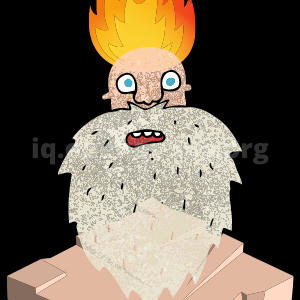×

Search anything:

# 100+ Graph Algorithms and Techniques [Complete List]

#### Graph Algorithms Algorithms CultureGet this book -> Problems on Array: For Interviews and Competitive Programming

In this article, we have listed 100+ problems on Graph data structure, Graph Algorithms, related concepts, Competitive Programming techniques and Algorithmic problems. You should follow this awesome list to master Graph Algorithms.

There are different categories of problems like Topological Sorting, Shortest Path in Graph, Minimum Spanning Tree, Maximum Flow Problem, Graph Coloring Problem, Maximum Matching Problem and much more.

### Basics of Graph Algorithms

These are basic ways to represent a Graph and basic traversal techniques which you must learn about before attempting challenging problems.

### Topological Sorting: order nodes in a Graph

Topplogical Sort is an important technique to order nodes in a Graph based on dependencies. It is used to solve several important problems.

### Shortest Path in Graph

Find the shortest path between two nodes in a Graph is a very important problem and several algorithms exist.

Some basic path related algorithms before finding the shortest path:

Standard algorithms to find shortest path:

### Minimum Spanning Tree

Minimum Spanning Tree is a subset of a graph that connects all nodes by minimizing the cost of connecting edges. This is useful in solving several key problems.

### Maximum Flow Problem

Maximum Flow is an important graph problem with applications in Physics and Engineering.

### Graph Coloring Problem

Graph Coloring is a key problem and is used to solve a wide range of challenging problems.

### Maximum Matching Problem

Maximum Matching is a problem of pairing nodes with a constraint and is important for solving a vast range of problems.

Understanding pairing nodes in Graphs (Maximum Matching): Basics of Maximum Matching
Hopcroft Karp algorithm: This technique takes O(E V0.5) time.
Hungarian Maximum Matching Algorithm: This original algorithm took O(V4) time while an optimized version takes O(V3) time.
Blossom Maximum Matching Algorithm: This technique takes O(E V2) time while a much more complex variant of it takes O(E V0.5) time.

### Stable Marriage Problem

Stable Marriage Problem is variant of Maximum Matching problem and is used in real life problems.

Basics of stable matching: Overview of Stable Marriage Problem and related concepts.
Gale Shapley Algorithm for Stable Matching problem: Time Complexity of this technique is O(V2) time.
Stable Roommates Problem (Irving's Algorithm): Time Complexity of this technique is O(V2) time.
Variants of Stable Marriage Problem: There are several variants of Stable Marriage Problem
Hospital Residents Problem:

### Maximum / Minimum Cut Problems

Minimum and Maximum cut problems are important problem as it reveals the weakest problem in a graph. In real problem, these algorithms are used to find weak points and fix them / or prepare for it.

Overview of Minimum Cut Problem: Overview of Minimum Cut Problem
Overview of Maximum cut problem: Overview of Maximum Cut Problem
Find cut edges in a graph: An algorithm to find cut edges in a graph.
Find articulation points or cut vertices in a graph: An algorithm to find cut vertices (not edges) in a graph.
Find articulation point in Graph: An algorithm to find articulation point in a graph.

### Strongly Connected Components

Strongly Connected Components are sub-graphs where every vertex is connected by a path.

### Travelling Salesman Problem

Travelling Salesman Problem is a NP-Complete problem and is one of the most difficult problems in Computer Science.

### Other Graph based Problems

These are other core Graph based problems that you must learn about.

### Applications of Graph in Real problems

With this article at OpenGenus, you must have a strong hold of Graph data structure, related concepts and Algorithmic problems based on it. Keep practicing.

Try learning other algorithmic concepts: